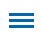Building fluency with addition and subtraction is a critical area of focus for second-grade math instruction. In addition to mastery of basic facts within 20, skip counting and other computations strategies learned from previous grades are applied. Students’ use of computation skills coincides with the ability to solve word problems involving money. The four-page Post Office Problems template requires students to apply these skills to present a handful of stamp-buying-related questions.

Editor's Note: This blog is the second in a series of posts from former math specialist Scott Loomis on the real world math templates he has created for Wixie and how you can use and modify them for your own awesome performance tasks. You can read the backstory on his experience and expertise below.

## Post Office Problems

Instructional Concept: Solve word problems involving money.Location: Curriculum Library > Math > Measurement > Money

Estimated Completion Time: 15-20 minutes

## Common Core State Standards for Mathematics

CCSS.MATH.CONTENT.2.MD.C.8
Measurement and Data
Work with time and money.
Solve word problems involving dollar bills, quarters, dimes, nickels, and pennies, using \$ and ¢ symbols appropriately. Example: If you have 2 dimes and 3 pennies, how many cents do you have?

CCSS.MATH.CONTENT.2.NBT.B.5
Number & Operations in Base Ten
Use place value understanding and properties of operations to add and subtract.
Fluently add and subtract within 100 using strategies based on place value, properties of operations, and/or the relationship between addition and subtraction.

CCSS.MATH.CONTENT.2.OA.A.1
Operations & Algebraic Thinking
Represent and solve problems involving addition and subtraction.
Use addition and subtraction within 100 to solve one- and two-step word problems involving situations of adding to, taking from, putting together, taking apart, and comparing, with unknowns in all positions, e.g., by using drawings and equations with a symbol for the unknown number to represent the problem.

Topics: Wixie, math, real world of math